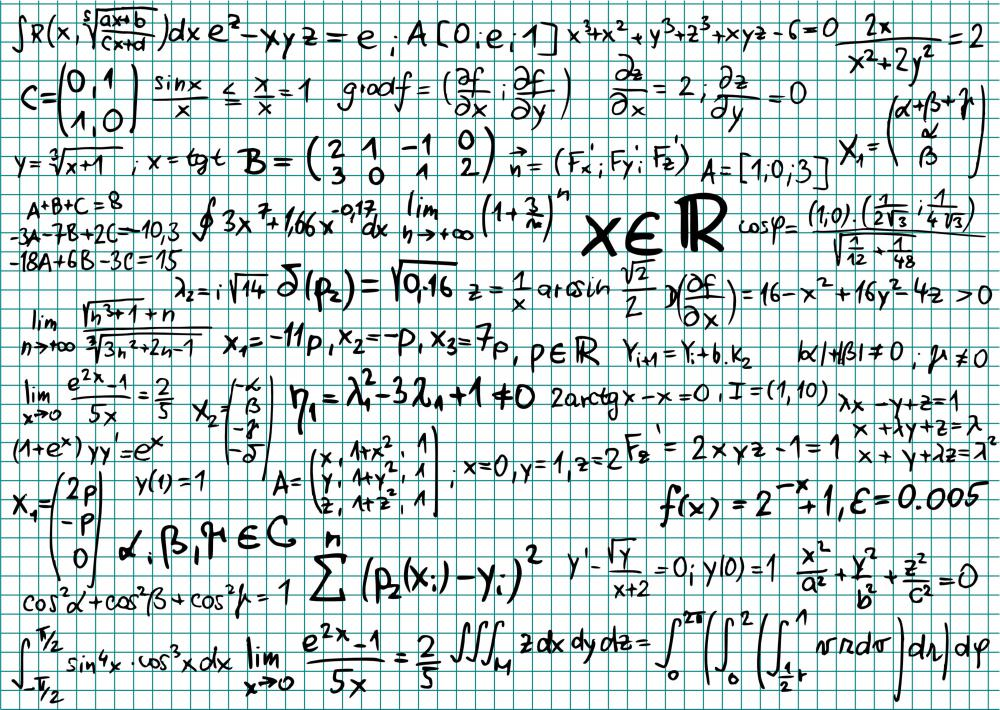# What Is a Canonical Form?

David Isaac Rudel
David Isaac RudelMathematicians find canonical forms particularly useful.

Almost all mathematical objects can be expressed in multiple ways. For example, the fraction 2/6 is equivalent to 5/15 and -4/-12. A canonical form is a specific schema that mathematicians use to describe objects from a given class in a codified, unique way. Every object in the class has a single canonical representation matching the template of the canonical form.A canonical form is a specific schema that mathematicians use to describe objects from a given class in a codified, unique way.

For rational numbers, the canonical form is a/b, where a and b have no common factors and b is positive. Such a fraction is typically described as being “in lowest terms.” When put into canonical form, 2/6 becomes 1/3. If two fractions are equal in value, their canonical representations are identical.

Canonical forms are not always the most common way of denoting a mathematical object. Two-dimensional linear equations have the canonical form Ax + By + C = 0, where C is either 1 or 0. Yet mathematicians often employ the slope-intercept form — y = mx + b — when doing basic calculations. The slope-intercept form is not canonical; it cannot be used to describe the line x = 4.

Mathematicians find canonical forms particularly useful when analyzing abstract systems, in which two objects might seem markedly different but are mathematically equivalent. The set of all closed paths on a doughnut has the same mathematical structure as the set of all ordered pairs (a, b) of integers. A mathematician can see this connection easily if he uses canonical forms to describe both sets. The two sets have the same canonical representation, so they are equivalent. To answer a topological question about curves on a doughnut, a mathematician might find it easier to answer an equivalent, algebraic question about ordered pairs of integers.

Many fields of study employ matrices to describe systems. A matrix is defined by its individual entries, but those entries frequently do not convey the character of the matrix. Canonical forms help mathematicians know when two matrices are related in some way that might not be obvious otherwise.

Boolean algebras, the structure that logicians use when describing propositions, have two canonical forms: disjunctive normal form and conjunctive normal form. These are algebraically equivalent to the factoring or expansion of polynomials respectively. A short example illustrates this connection.

The principal of a high school might say, “The football team must win one of its first two games and beat our rivals, the Hornets, in its third game, or else the coach will be fired.” This claim can be written logically as (w1 + w2) * H + F, where “+” is the logical “or” operation and “*” is the logical “and” operation. The disjunctive normal form for this expression is w1 *H + w2 *H + F. Its conjunctive normal form for is (w1 + w2 + F) * (H + F). All three of these expressions are true under exactly the same conditions, so they are logically equivalent.

Engineers and physicists also make use of canonical forms when considering physical systems. Sometimes one system will be mathematically similar to another even though they appear nothing alike. The differential matrix equations that are used to model one might be identical to those used to model the other. These similarities become apparent when the systems are cast in a canonical form, such as observable canonical form or controllable canonical form.

## You might also Like## Discuss this Article

Forgot password?
###### Register:
•Mathematicians find canonical forms particularly useful.
•A canonical form is a specific schema that mathematicians use to describe objects from a given class in a codified, unique way.